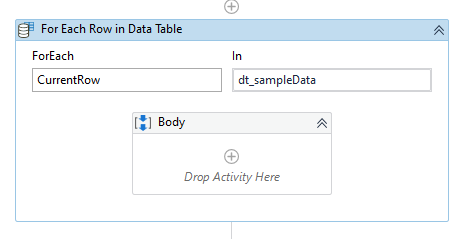# How to get specific data from data table and make it as value to be typed in TypeInto activity

Hi,

Would like to ask for your help on how will i be able to get the specific value in the data table and put it as value in the TypeInto activity? Because I’m getting an error that every loop. I always the the value in data row ‘0’.

Thank you!

Hi @_waysi,

Can you share the scenario with screenshot which would be helpful to suggest the best method.

Regards,
@90s_Developer

Hi @_waysi ,

If you are using it inside of a For Each Row in DataTable Activity, then you can use this:

``````CurrentRow(0).ToString
``````

If its a DataTable that you are directly referencing, then you need to know which column it resides it:
dt.Rows(rowIndex)(columnIndex)

``````dt.Rows(0)(0).ToString
``````

Kind Regards,
Ashwin A.K

Thanks for the reply.

Here is the scenario.

I have a tsv file with data and it was stored in a data table.

Sample is.
Column1 Column2 Column3
AA AA1 AA2
BB BB1 BB2
CC CC1 CC2

It ia stored in sample_dt

Then I have a do while activity where in i need to input data in a field.

In the first loop:
The values that will be getting is the datarow(0)
TextField1 = AA
TextField2 = AA1
TextField3 = AA2

Then it will be save the next loop the data to be inputted ahould be the one in datarow(1)
TextField1 = BB
TextField2 = BB1
TextField3 = BB2

Then same goes to the last loop.

But in my current code, in the second loop. I still get the data from datarow(0).

Is this enough? Thanks!

Is this applicable for this scenario?

Hi @_waysi ,

When you reference CurrentRow(0).ToString in the first iteration, it will retrieve data from the First Row from the First Column i.e.,

Column1 Column2 Column3
AA AA1 AA2
BB BB1 BB2
CC CC1 CC2

Similarly, CurrentRow(1).ToString will retrieve data from the First Row and the Second Column

Column1 Column2 Column3
AA AA1 AA2
BB BB1 BB2
CC CC1 CC2

I hope we are on the same page here?

Kind Regards,
Ashwin A.K

Is CurrentRow(n) can be put inside the Type Into activity? Or should I declare it first?

Hi @_waysi ,

Its better if you use a For Each Row in DataTable Activity, the CurrentRow variable is an auto-generated local variable.Kind Regards,
Ashwin A.K

1 Like

Please find the below attached screenshots for your reference.

1. Using For Each Row in DataTable method
2. Without Using For Each Row in DataTable methodAttached the sample workflow XAMLfor your reference.

Hope this method solves your requirement.

FetchingValuefromDatatable.xaml (11.6 KB)

Regards,
@90s_Developer

1 Like

The variable CurrentRow indicates one row of that particular DataTable. So, for each iteration, the rows are called one by one.

`Syntax: CurrentRow(index).ToString`

Here, index indicates the column number.
So, the starting index would be 0 which indicates the values present in the Column A and sameway for Column B the index will be 1

If your value is present in the 4th column, the CurrentRow(3).ToString

Regards,
@90s_Developer

1 Like

It works but what if I want to enter first and second row to the grid? How will I proceed to next row?

It works but what if I want to enter first and second row to the grid? How will I proceed to next row? Thanks!

Here is the scenario.

I have a tsv file with data and it was stored in a data table.

Sample is.
Column1 Column2 Column3
AA AA1 AA2
BB BB1 BB2
CC CC1 CC2

It ia stored in sample_dt

Then I have a do while activity where in i need to input data in a field.

In the first loop:
The values that will be getting is the datarow(0)

First row will get the:
Column1=AA
Column2 = AA1
Column3 = AA2

Then it will go to second row with:
Column1=BB
Column2 = BB1
Column3 = BB2

Then another loop for CC values.

Using foreach how will I be able to step in the next row?

Thank you!

Here is the scenario.

I have a tsv file with data and it was stored in a data table.

Sample is.
Column1 Column2 Column3
AA AA1 AA2
BB BB1 BB2
CC CC1 CC2

It ia stored in sample_dt

Then I have a do while activity where in i need to input data in a field.

In the first loop:
The values that will be getting is the datarow(0)

First row will get the:
Column1=AA
Column2 = AA1
Column3 = AA2

Then it will go to second row with:
Column1=BB
Column2 = BB1
Column3 = BB2

Then another loop for CC values.

Using foreach how will I be able to step in the next row?

Thanks!

As I mentioned before, refer this thread.

For Each Row in DataTable will loop through each row by row of the datatable.
So, in the `CurrentRow(index)`, here the index plays the role of column i.e., it indicates which column’s row.

For example, in a datatable there are five columns Column1, Column2…Column5 and has 10 rows totally. Need to fetch the row values only from Column3 then `CurrentRow(2).ToString` which will print all the values present in the column3 alone.
Note: While reading the excel file using Read Range, make sure you enable the AddHeaders property in the properties panel of the Read Range activity.

Hope this helps you to solve your issue.

Regards,
@90s_Developer

1 Like

This topic was automatically closed 3 days after the last reply. New replies are no longer allowed.